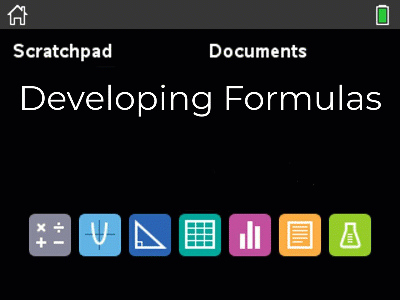•• ##### Device
•TI-Nspire™ CX

TI-Nspire™

5.0

# QLD (Nspire): Induction Part 1 - Whole Numbers

by Texas Instruments#### Objectives

Part 1 of 3: Induction - students build equations visually, graphically and numerically and then prove their formula to be true for all whole number by mathematical induction. Additional questions are provided for students to practice the process of mathematical induction.

#### Vocabulary

• Induction
• Sum
• Cumulative sum